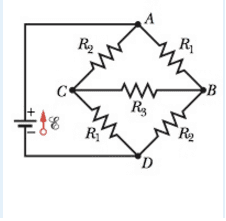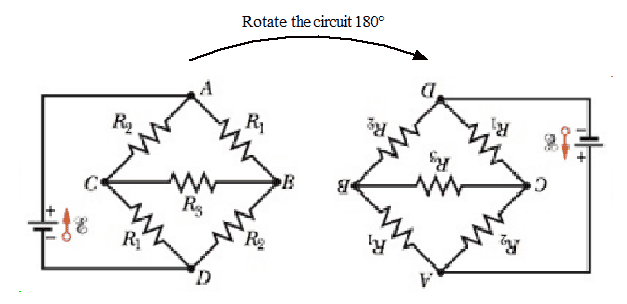# Kirchoff's method for a weird circuit

• Sho Kano
In other words, the current and potential difference remains the same, it's just that they are now pointing in the opposite direction.Since the currents through R2 are the same, and the currents through R1 are the same because they are in the opposite polarities, it follows that the current through R2 is also the same. In summary, the current through resistor R2 is 2000 Ω.

## Homework Statement

In the following circuit, the battery has emf ε = 12.7 V. The resistors are R1 = 2000 Ω . R2 = 3000 Ω, and R3 = 4000 Ω. What is the current through resistor R2 ?

Kirchoff's Rules
V=IR

## The Attempt at a Solution

i0 (into A) - i2 (current into resistor 2) - i3 (current into resistor 3) = 0
Then what happens at C and B?

For some reason the image failed to upload. Here it isSho Kano said:

## Homework Statement

In the following circuit, the battery has emf ε = 12.7 V. The resistors are R1 = 2000 Ω . R2 = 3000 Ω, and R3 = 4000 Ω. What is the current through resistor R2 ?

Kirchoff's Rules
V=IR

## The Attempt at a Solution

i0 (into A) - i2 (current into resistor 2) - i3 (current into resistor 3) = 0
Then what happens at C and B?
There may be simpler ways to solve this, but just go ahead and write the KCL equations and try it that way. Please show your work...Here is a thread with a similar circuit.

berkeman said:
There may be simpler ways to solve this, but just go ahead and write the KCL equations and try it that way. Please show your work...i0 = initial current
i1 = current to R2
i2 = current to R1
i3 = current to R3
i4 = current to lower R1
i5 = current to lower R2

i0 - i1 - i2 = 0
i3 + i1 - i4 = 0
i2 - i3 - i5 = 0
-i2R1 - i3R3 + i1R2 = 0
-i5R2 + i4R1 + i3R3 = 0
12.7 - i1R2 - i4R1 = 0

Last edited:
The currents are as follows from i0 to i5
.0052
.0024
.0028
.0004
.0028
.0024
Since the ones through R1 and R2 are identical, it does seem like there is an easier way to solve this, but how?

Sho Kano said:
Since the ones through R1 and R2 are identical, it does seem like there is an easier way to solve this, but how?
Did you read through the thread that SammyS linked to in post #4?Sho Kano said:
i0 = initial current
i1 = current to R2
i2 = current to R1
i3 = current to R3
i4= current to lower R1 = i2 + i3
i5= current to lower R2 = i1 - i3
It is usually preferable to not involve more current variables than are needed, to minimise the amount of algebra it generates. Once you label 3 currents, the others are already defined in terms of those 3 so they can be marked on the schematic accordingly.

NascentOxygen said:
It is usually preferable to not involve more current variables than are needed, to minimise the amount of algebra it generates. Once you label 3 currents, the others are already defined in terms of those 3 so they can be marked on the schematic accordingly.
So in the end, I only need 5 equations?

12.7 - i1R2 - (i1 + i3)R1 = 0
-i2R1 - i3R3 + i1R2 = 0
i3R3 - (i1+i3)R2 + i5R1 = 0
i0 - i1 - i2 = 0
i2 - i3 - i5 = 0 or i1 + i3 - i2 = 0

Last edited:
berkeman said:
Did you read through the thread that SammyS linked to in post #4?Yes, the currents through R2 are the same and so are the ones for R1 because reversing the polarities would give an identical result.

So that means i4 = i2 and i1 = i5
i0 - i1 - i2 = 0
i2 - i3 - i1 = 0 or i1 + i3 - i2 = 0
12.7 - i1R2 - i2R1 = 0
-i2R1 - i3R3 + i1R2 = 0
i3R3 - i1R2 + i2R1 = 0
?

But I'm not all convinced about the reversing polarities proof. Who's to say that once the current passes through the bridge and all the other junctions, it's the same?

Last edited:
Sho Kano said:
But I'm not all convinced about the reversing polarities proof. Who's to say that once the current passes through the bridge and all the other junctions, it's the same?
Symmetry says so. If you can flip or rotate a circuit about one or more axes of symmetry and the result is identical to the original circuit, then the resulting circuit must behave identically to the original and the symmetric pairs of circuit elements must display the same behavior (current, potential difference).

Take your original circuit and rotate it 180°. Doing so cannot change the circuit behavior since no connections have changed (the topology remains the same).Note that the bridge circuit still has the same values of resistance in all the same locations. The potential supplied by the voltage source has the opposite polarity in this view (negative at top rather than positive at top), but the polarity that we assign + and - potentials and current directions are just a matter of convention. You can change from assuming positive charge carriers to negative charge carriers without affecting any equations. So the symmetry pairs of resistances must carry the same currents in both orientations.

•berkeman
i0 - i1 - i2 = 0
i3 + i1 - i4 = 0
i2 - i3 - i5 = 0
-i2R1 - i3R3 + i1R2 = 0
-i5R2 + i4R1 + i3R3 = 0
12.7 - i1R2 - i4R1 = 0
gneill said:
Symmetry says so. If you can flip or rotate a circuit about one or more axes of symmetry and the result is identical to the original circuit, then the resulting circuit must behave identically to the original and the symmetric pairs of circuit elements must display the same behavior (current, potential difference).

Take your original circuit and rotate it 180°. Doing so cannot change the circuit behavior since no connections have changed (the topology remains the same).
View attachment 99483

Note that the bridge circuit still has the same values of resistance in all the same locations. The potential supplied by the voltage source has the opposite polarity in this view (negative at top rather than positive at top), but the polarity that we assign + and - potentials and current directions are just a matter of convention. You can change from assuming positive charge carriers to negative charge carriers without affecting any equations. So the symmetry pairs of resistances must carry the same currents in both orientations.
Are you trying to say that the current into point A for the non-rotated circuit is the same as the current flowing into point D for the rotated circuit?

Sho Kano said:
i0 - i1 - i2 = 0
i3 + i1 - i4 = 0
i2 - i3 - i5 = 0
-i2R1 - i3R3 + i1R2 = 0
-i5R2 + i4R1 + i3R3 = 0
12.7 - i1R2 - i4R1 = 0

Are you trying to say that the current into point A for the non-rotated circuit is the same as the current flowing into point D for the rotated circuit?
The magnitude is certainly the same. Change the polarity of the voltage source and the signs of the currents will agree too.

Besides, how could they be different, since the same current that leaves the battery must return to it?

gneill said:
The magnitude is certainly the same. Change the polarity of the voltage source and the signs of the currents will agree too.

Besides, how could they be different, since the same current that leaves the battery must return to it?
Right, charge is conserved. If all the resistors were different, would the current still be the same?

Sho Kano said:
Right, charge is conserved. If all the resistors were different, would the current still be the same?
Which current? Certainly the current entering (or leaving) node A via the battery is the same as the current leaving (or entering) node D via the battery.

gneill said:
Which current? Certainly the current entering (or leaving) node A via the battery is the same as the current leaving (or entering) node D via the battery.
I see, so only currents through same resistors will have the same magnitude?

Sho Kano said:
I see, so only currents through same resistors will have the same magnitude?
Rephrase that as "The currents through the symmetrically paired resistors will be the same" and you would be right. Don't overspecify by saying "only". Some circuits may have resistors of different values that happen to have the same magnitude of current.

gneill said:
Rephrase that as "The currents through the symmetrically paired resistors will be the same" and you would be right. Don't overspecify by saying "only". Some circuits may have resistors of different values that happen to have the same magnitude of current.
The currents through the symmetrically paired resistors will be the same.
What if the resistors have different resistances?

Sho Kano said:
The currents through the symmetrically paired resistors will be the same.
What if the resistors have different resistances?
Will there still be the same symmetry?

Precisely which resistances will you change?

SammyS said:
Will there still be the same symmetry?

Precisely which resistances will you change?
In the un-rotated figure: top left resistor is R1 top right is R2. Bottom left is R3, bottom right is R4. The bridge resistor is R5.

Sho Kano said:
The currents through the symmetrically paired resistors will be the same.
What if the resistors have different resistances?
Then the currents will be affected. No more assumptions about one current being the same as another.